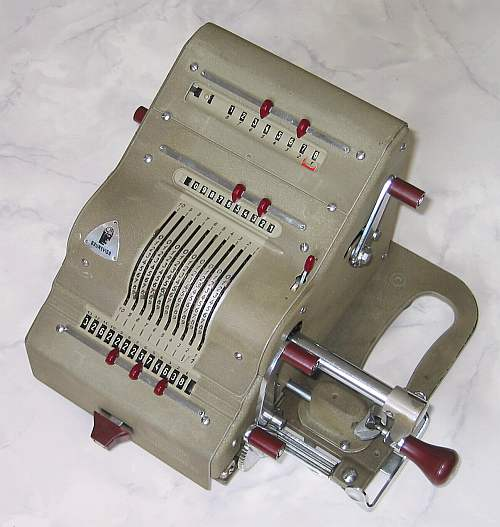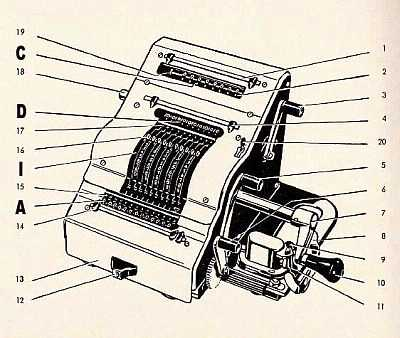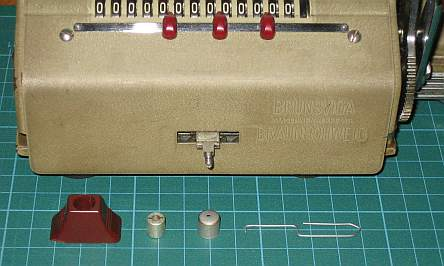previous <<==>> next

BRUNSVIGA-13RK``` TECHNICAL DATA of the Mechanical Calculator BRUNSVIGA-13RK ************************************************************************** Serial Number: ??? ( missing plaque ) Dimensions: (ca.) Width = 11_1/2" / 29 cm Depth = 9 " / 23 cm Height = 6_1/4" / 16 cm Weight: (ca.) 18 lbs / 8 kg Mechanics: Pin-Wheel / Sprossenrad 10s Carry Mechanism Functions: Add, Subtract, Multiply, Divide Back-Transfer / Rueckuebertragung Registers: Input = 10 Decimals Counter = 8 Decimals Arithmetic = 13 Decimals Manufacturer: BRUNSVIGA MASCHINENWERKE AG Braunschweig West Germany 1950 `````` H O W T O U S E the BRUNSVIGA-13RK ... ************************************************************************** Make sure that the TRANSPORTATION-LOCK under [ A ] is opened !!! BASIC SETTINGS: =============== (a) Crank: ---------- For addition (+) the crank  is turned clockwise; for subtraction (-) the crank is turned counter-clockwise. In the idle position  the crank is down & locked. To make one or more turns with the crank, pull out the handle  to unlock. When finished the turn(s), let the handle snap into lock again. The locked down position only makes other functions accessible. REMARK: Every started turn has to be finished completely! Accidentally started turns are correctible somehow. (b) Clearing Registers: ----------------------- The input [I] register is manipulated by 10 levers. Its content is shown in the display [D]. The input can be cleared with the lever  on the left side. The arithmetic [A] register is to clear with the small lever  on the right side. Levers [3 & 5] are working together with the switch  on top. If switch  is in down (.) position: Lever  clears the counter [C] only; Lever  clears arithmetic & counter [A & C]. If switch  is in up (..) position: Lever  clears counter & input [C & I]; Lever  clears all registers [A & C & I]. R E M A R K : Windows of counter, input & arithmetic show a RED INDICATOR in the PROCESS of CLEARING. (c) Shifting the Carriage: -------------------------- With the 2-grip lever  (located under the crank) the carriage  is movable one step right or one step left. Also with the front button  either right or left - but additional continuously when pressed forward and right or left. The left position "1" is the "Start Position" of the carriage. (d) Counting Direction: ----------------------- After clearing the counter [C] a white mark  shows its neutral state. It depends of the 1st turn of the crank what happens: When (for addition) the crank's 1st turn is clockwise, the counter display  shows white figures; when (for subtraction) the crank's 1st turn is counter-clockwise, the counter display  shows red figures. In both cases the counter is working with 10s-carry - and allows "Shortened Method of Multiplication". A red cursor moves over the counter's display and shows the active position refered to the carriage's position. (e) Back Transfer: ------------------ For continuous multiplication the contents of the arithmetic unit can be back transfered into the input register with this procedure: Pull & HOLD lever ; pull lever , release , RELEASE  A ==>> I ; A & C cleared ADDITION & SUBTRACTION: ======================= Example: 123 + 45 - 6 = 162 Clear input, counter and arithmetic units. Carriage in position 1. ADD: Enter the first number (123) in the far right of the input unit. Make a positive (clockwise) turn with the crank to transfer the number into arithmetic unit. The counting unit displays the figure 1. Enter the second number (45). Make a positive (clockwise) turn with the crank to add the number. The arithmetic unit displays the intermediate sum (168) and the counting unit displays the figure 2. SUBTRACT: Enter the third number (6). Make a negative (counter-clockwise) turn with the crank. The arithmetic unit displays the result (162) and the counting unit is decreased by 1. REMARK: NEGATIVE RESULTS are displayed in the arithmetic unit as the COMPLEMENT of the next higher 10, 100, 1000, ... Example: -12 = 99...9988 MULTIPLICATION: =============== Example: 123 x 45 = 5535 Clear input, counter and arithmetic units. Enter the multiplicand (123) in the far right of the input unit. The multiplicator (45) has two digits, so the carriage is shifted to position 2 by pressing the 2-grip lever  forward. Make positive (clockwise) turns with the crank, until the first figure of the multi- plicator (4) will appear in the 2nd position of the counter unit. Shift the carriage to position 1 by pressing the 2-grip lever  backward. Repeat making positive turns with the crank, until the second figure of the multiplicator (5) appears in the 1st position of the counter unit. The multiplication is done: The multiplicand (123) stays in the input unit, the multiplicator (45) in the counter and the result (5535) is in the arithmetic unit. DIVISION: ========= Example: 22 : 7 = 3.1428571 Remainder 3 Division requires 3 steps: (1st) To Set the Dividend into Arithmetic Unit: ----------------------------------------------- For the maximum number of decimals, pull out the carriage to the far right position. Enter the dividend (22) in the far right of the input unit. Make a positive (clockwise) turn with the crank to transfer into arithmetic unit. (2nd) To Set the Divisor into Input Unit: ----------------------------------------- Clear counter & input units. Enter the divisor (7) above the dividend (22). (3rd) To Divide: ---------------- Make negative (counter-clockwise) turns (the counter will now show red figures!) with the crank until the arithmetic unit shows an "underflow". Make one positive (clockwise) turn with the crank. Move the carriage to the next left position. Repeat this procedure until the required number of decimals are calculated... The result (3.1428571) is in the counter unit, and the remainder (3) is in the arithmetic unit. The divisor (7) stays in the input unit, therefore an additional decimal can be estimated... ( 4, cause 4 x 7 = 28). Have a look at "Calculating Trickies" ... HOW TO CLEAN THE A R I T H M E T I C UNIT OF THE BRUNSVIGA-13RK ??? ========================================================================== Bent & Cut a PaperClip appropriate to PULL-OUT the METAL COVER in  ...... THAN A NUT is accessable ... and the COVER OF [ A ] CAN BE REMOVEDED. impressum: ************************************************************************** © C.HAMANN http://public.BHT-Berlin.de/hamann 08/15/12 ```